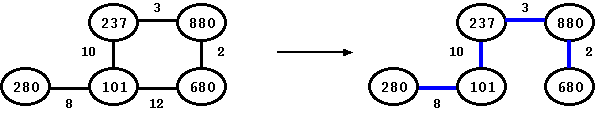CodingBison

In this section, we discuss two algorithms that build minimum spanning tree for weighted graphs: Prim's algorithm and Kruskal's algorithm. A minimum spanning tree is a collection of the minimum number of edges such that it connects all the vertices and the cumulative weight of all of these edges is the minimum.

There are many uses of minimum spanning tree. For example, if we were to travel to all the vertices of the graph, then using the selected edges from the selected minimum spanning tree would have the least weight. This can be used in many applications, like doing multicast in the network.

With that, let us see an example. In the following picture, we have a graph with 5 vertices and 5 edges. Each edge has a weight attached to it. If we were to build a minimum spanning tree, then we would need to select all the edges except 101-680. With that the total weight of the tree would be 8+10+3+2 or 25. Thus, this is the tree that has the total minimum weight for all edges.Figure: Building a Minimum Spanning Tree

Prim's algorithm is based on breadth-first-search. It builds a minimum spanning tree by continuously adding newer vertices to it, one at each step. The tree starts by selection of a vertex (which is the root of the tree) and then it keeps adding a new vertex to the existing tree. The new vertex that is added is the one that has the least weight among all the vertex that are connected to the tree.

Kruskal's algorithm uses set-based data structure to build the minimum spanning tree. It starts by adding all vertices as long as they belong to different sets. In this algorithm, edges are selected based on their weight and so it does not maintain a tree at each step. Instead, at each step, it adds a new edge that has the least weight. This leads to edges being added such that they can form a forest and not tree. What this also means is that if the input graph is not connected (let us say, it has K partitions), then Kruskal's algorithm would generate K trees, one per partition. Prim's algorithm would not be able to advance beyond the partition that has the starting vertex (aka the root of the Prim's tree).

Building minimum spanning trees are different from shortest path algorithm like Dijkstra's algorithm. In Dijkstra's algorithm, our goal is to build an array that provides the shortest distance of all nodes from a given (source) node. In minimum spanning tree, the goal is to build a tree such that the sum total of all of the edges is the minimum.